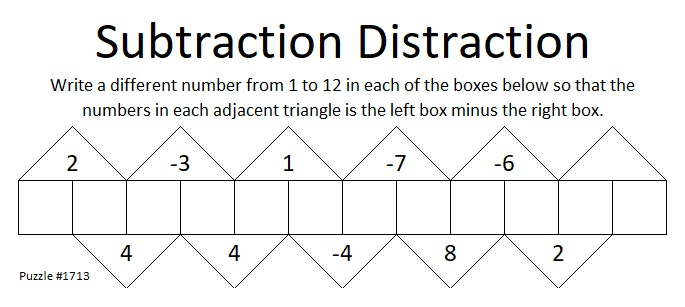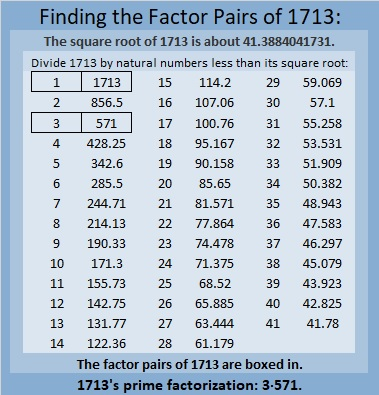# 1713 A Little More of a Subtraction Distraction

Contents

### Today’s Puzzle:

It occurred to me that as long as the last box is neither 1 nor 12 that I could leave the clue above it blank. Can you still solve the puzzle?### Factors of 1713:

• 1713 is a composite number.
• Prime factorization: 1713 = 3 × 571.
• 1713 has no exponents greater than 1 in its prime factorization, so √1713 cannot be simplified.
• The exponents in the prime factorization are 1 and 1. Adding one to each exponent and multiplying we get (1 + 1)(1 + 1) = 2 × 2 = 4. Therefore 1713 has exactly 4 factors.
• The factors of 1713 are outlined with their factor pair partners in the graphic below.### More About the Number 1713:

1713 is the difference of two squares in two different ways:
857² – 856² = 1713, and
287² – 284² = 1713.

1713 is the sum of two, three, and six consecutive numbers:
856 + 857 = 1713,
570 + 571 + 572 = 1713, and
283 + 284 + 285 + 286 + 287 + 288 = 1713.

Do you see any relationship between those two facts?

This site uses Akismet to reduce spam. Learn how your comment data is processed.